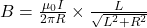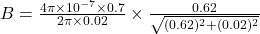## A straight wire of length 0.62 m carries a conventional current of 0.7 amperes. What is the magnitude of the magnetic field made by the curr

Question

A straight wire of length 0.62 m carries a conventional current of 0.7 amperes. What is the magnitude of the magnetic field made by the current at a location 2.0 cm from the wire

in progress 0
5 months 2021-09-03T18:01:32+00:00 1 Answers 2 views 0

Magnetic field at point having a distance of 2 cm from wire is 6.99 x 10⁻⁶ T

Explanation:

Magnetic field due to finite straight wire at a point perpendicular to the wire is given by the relation :……(1)

Here I is current in the wire, L is the length of the wire, R is the distance of the point from the wire and μ₀ is vacuum permeability constant.

In this problem,

Current, I = 0.7 A

Length of wire, L = 0.62 m

Distance of point from wire, R = 2 cm = 2 x 10⁻² m = 0.02 m

Vacuum permeability, μ₀ = 4π x 10⁻⁷ H/m

Substitute these values in equation (1).B = 6.99 x 10⁻⁶ T